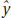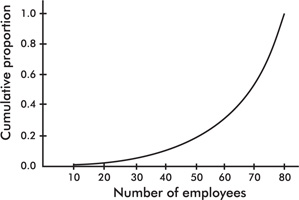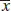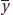# AP Statistics Multiple-Choice Practice Test 33

### Test Information10 questions23 minutes

1. A company wishes to determine the relationship between the number of days spent training employees and their performances on a job aptitude test. Collected data result in a least squares regression line,= 12.1 + 6.2x, where x is the number of training days andis the predicted score on the aptitude test. Which of the following statements best interprets the slope and y-intercept of the regression line?

2. To survey the opinions of the students at your high school, a researcher plans to select every twenty-fifth student entering the school in the morning. Assuming there are no absences, will this result in a simple random sample of students attending your school?

3. Consider a hypothesis test with H0 : μ = 70 and Ha : μ < 70. Which of the following choices of significance level and sample size results in the greatest power of the test when μ = 65?

4. Suppose that 60% of a particular electronic part last over 3 years, while 70% last less than 6 years. Assuming a normal distribution, what are the mean and standard deviation with regard to length of life of these parts?

5. The label on a package of cords claims that the breaking strength of a cord is 3.5 pounds, but a hardware store owner believes the real value is less. She plans to test 36 such cords; if their mean breaking strength is less than 3.25 pounds, she will reject the claim on the label. If the standard deviation for the breaking strengths of all such cords is 0.9 pounds, what is the probability of mistakenly rejecting a true claim?

6. The graph below shows cumulative proportions plotted against numbers of employees working in mid-sized retail establishments.What is the approximate interquartile range?

7. What is a placebo?

8. To study the effect of alcohol on reaction time, subjects were randomly selected and given three beers to consume. Their reaction time to a simple stimulus was measured before and after drinking the alcohol. Which of the following is a correct statement?

9. Suppose that the regression line for a set of data,= 7x + b, passes through the point (-2, 4). Ifandare the sample means of the x- and y-values, respectively, then=

10. Which of the following is a false statement about simple random samples?